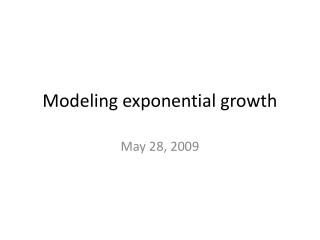Download PresentationModeling exponential growth

# Modeling exponential growth - PowerPoint PPT PresentationDownload Presentation## Modeling exponential growth

- - - - - - - - - - - - - - - - - - - - - - - - - - - E N D - - - - - - - - - - - - - - - - - - - - - - - - - - -
##### Presentation Transcript

1. Modeling exponential growth May 28, 2009

2. Objectives • Use exponential functions to model exponential growth and decay

3. Parts of an exponential function y=bxis the model that we discussed yesterday. When the initial value (the value at zero) isn’t one, new model is: y=a∙bx a is the initial value b is the growth rate (or decay rate)

4. Modeling exponential growth • To model exponential growth, determine the original amount (a). • Then determine the percent increase. Change this into a decimal and add it to 1. This is the growth rate (b). • Plug in the number of years, months, days, etc. (x). I have 4 weasels. Their growth rate is 150% per year. How many weasels will I have after 10 years?

5. Try this one A certain species of plant grows at the rate of 14% per week. If you began with 100 plants, how many will you have after 3 weeks? A month? A year?